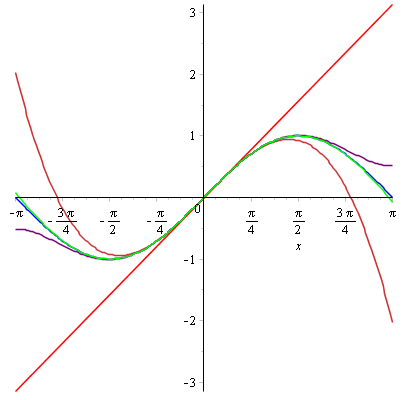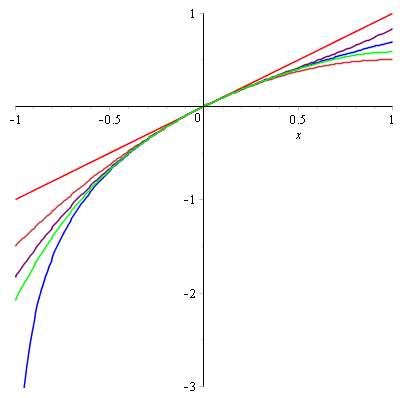# Maclaurin and Taylor series

## Introduction

Suppose that a function $f(x)$ can be expressed as the polynomial

$$f(x) = a_0 + a_1 x + a_2 x^2 + a_3 x^3 + \ldots + a_k x^k + \ldots$$

where $a_i$ are constant coefficients. Then

\begin{align} f'(x)&= a_1 + 2a_2 x+3a_3 x^2 + \ldots \\ f''(x) &= 2a_2 + 6a_3 x + \ldots \\ f'''(x) &= 6a_3 + \ldots \end{align}

And so on. Then

\begin{align} f(0) &= a_0 \\ f'(0) &= a_1 \\ f''(0) &= 2a_2 \Rightarrow a_2 = \frac{f''(0)}{2} = \frac{f''(0)}{2!}\\ f'''(0) &= 6a_3 \Rightarrow a_3 = \frac{f'''(0)}{6} = \frac{f'''(0)}{3!} \end{align}

And so on. Then we can express $f(x)$ as

$$f(x) = f(0) + f'(0) x + \frac{f''(0)}{2!} x^2 + \frac{f'''(0)}{3!} x^3 + \ldots + \frac{f^{(k)}(0)}{k!} x^k + \ldots$$

This is the Maclaurin series for $f(x)$. Many differentiable functions can be expressed as their Maclaurin series. They are useful for approximating functions and finding approximate derivatives and integrals.

To find an approximation to $f(x)$ you truncate a function's Maclaurin series up to as many terms as you want. The more terms you use, the more accurate the approximation. Remember from the definition above that in theory, $f(x)$ is equal to the infinite Maclaurin series.

In FP2 you need to know the Maclaurin series expansions for $e^x$, $\sin x$, $\cos x$, and $\ln(1+x)$.

## Maclaurin series of $e^x$

Let $f(x) = e^x$. Then

\begin{align} f'(x) &= e^x \\ f''(x) &= e^x \\ f'''(x) &= e^x \\ & \ldots \\ f^{(k)}(x) &= e^x \end{align}

And so on. Then

\begin{align} a_0 &= f(0) = 1 \\ a_1 &= f'(0) = 1 \\ a_2 &= \frac{f''(0)}{2!} = \frac{1}{2!} \\ a_3 &= \frac{f'''(0)}{3!} = \frac{1}{3!} \\ & \ldots \\ a_k &= \frac{f^{(k)}(0)}{k!} = \frac{1}{k!} \end{align}

And so on. The formula for a Maclaurin series is

$$f(x) = f(0) + f'(0) x + \frac{f''(0)}{2!} x^2 + \frac{f'''(0)}{3!} x^3 + \ldots + \frac{f^{(k)}(0)}{k!} x^k + \ldots$$

Therefore the Maclaurin series of $e^x$ is

$$e^x \equiv 1 + x + \frac{x^2}{2!} + \frac{x^3}{3!} + \ldots + \frac{x^k}{k!} + \ldots$$

Here's a graph showing truncated version of the Maclaurin series compared to the actual $e^x$ graph.

• $y=e^x$ is in blue
• $y=1$ is in red
• $y=1 + x$ is in orange
• $y=1 + x + \frac{x^2}{2!}$ is in purple
• $y=1 + x + \frac{x^2}{2!} + \frac{x^3}{3!}$ is in greenSee how each progressively longer truncation gets closer to the $e^x$ graph.

## Maclaurin series of $\sin x$ and $\cos x$

Let $f(x) = \sin x$. Then $f(0) = 0$. Going through the derivatives

\begin{align} f'(x) &= \cos x \Rightarrow f'(0) = 1 \\ f''(x) &= -\sin x \Rightarrow f''(0) = 0 \\ f'''(x) &= -\cos x \Rightarrow f'''(0) = -1 \end{align}

And so on. The formula for a Maclaurin series is

$$f(x) = f(0) + f'(0) x + \frac{f''(0)}{2!} x^2 + \frac{f'''(0)}{3!} x^3 + \ldots + \frac{f^{(k)}(0)}{k!} x^k + \ldots$$

Therefore the Maclaurin series of $\sin x$ is

$$\sin x \equiv x - \frac{x^3}{3!} + \frac{x^5}{5!} - \frac{x^7}{7!} + \ldots + \frac{\left(-1\right)^k x^{2k+1}}{\left(2k+1\right)!} + \ldots$$

By similar reasoning the Maclaurin series of $\cos x$ is

$$\cos x \equiv 1 - \frac{x^2}{2!} + \frac{x^4}{4!} - \frac{x^6}{6!} + \ldots + \frac{\left(-1\right)^k x^{2k}}{\left(2k\right)!} + \ldots$$

Here's a graph showing truncated version of the Maclaurin series compared to the actual $\sin x$ graph.

• $y=\sin x$ is in blue
• $y=x$ is in red
• $y=x - \frac{x^3}{3!}$ is in orange
• $y=x - \frac{x^3}{3!} + \frac{x^5}{5!}$ is in purple
• $y=x - \frac{x^3}{3!} + \frac{x^5}{5!} - \frac{x^7}{7!}$ is in greenSee how each progressively longer truncation gets closer to the $\sin x$ graph.

Maclaurin series for trigonometric functions are particularly useful because many of them are periodic over $x$, and longer truncations provide extremely close approximations for relatively small $x$.

## Maclaurin series of $\ln(1+x)$

Let $f(x) = \ln(1+x)$. Then $f(0) = 0$. Going through the derivatives

\begin{align} f'(x) &= \frac{1}{1+x} \Rightarrow f'(0) = 1 \\ f''(x) &= -\frac{1}{\left(1+x\right)^{2}} \Rightarrow f''(0) = -1 \\ f'''(x) &= \frac{2}{\left(1+x\right)^{3}} \Rightarrow f'''(0) = 2 \\ f^{iv}(x) &= -\frac{6}{\left(1+x\right)^{4}} \Rightarrow f^{iv}(0) = -6 \end{align}

And so on. The formula for a Maclaurin series is

$$f(x) = f(0) + f'(0) x + \frac{f''(0)}{2!} x^2 + \frac{f'''(0)}{3!} x^3 + \ldots + \frac{f^{(k)}(0)}{k!} x^k + \ldots$$

Therefore the Maclaurin series of $\ln(1+x)$ is

$$\ln(1+x) \equiv x - \frac{x^2}{2} + \frac{x^3}{3} - \frac{x^4}{4} + \ldots + \frac{\left(-1\right)^{k-1} x^{k}}{k} + \ldots$$

This is valid for $|x|\lt 1$.

Here's a graph showing truncated version of the Maclaurin series compared to the actual $\ln(1+x)$ graph.

• $y=\ln(1+x)$ is in blue
• $y=x$ is in red
• $y=x - \frac{x^2}{2}$ is in orange
• $y=x - \frac{x^2}{2} + \frac{x^3}{3}$ is in purple
• $y=x - \frac{x^2}{2} + \frac{x^3}{3} - \frac{x^4}{4}$ is in greenSee how each progressively longer truncation gets closer to the $\ln(1+x)$ graph.

## Taylor series

Maclaurin series are actually a special case of Taylor series. Note that the Maclaurin series

$$f(x) = f(0) + f'(0) x + \frac{f''(0)}{2!} x^2 + \frac{f'''(0)}{3!} x^3 + \ldots + \frac{f^{(k)}(0)}{k!} x^k + \ldots$$

will not work for a function like $f(x)=\frac{1}{x}$ because $f(0)$ is undefined.

The Taylor series expansion for a function $f(x)$ is

$$f(x) = f(a) + f'(a) \left(x-a\right) + \frac{f''(a)}{2!} \left(x-a\right)^2 + \frac{f'''(a)}{3!} \left(x-a\right)^3 + \ldots + \frac{f^{(k)}(a)}{k!} \left(x-a\right)^k + \ldots$$

where $a$ is a non-zero constant. We say that this is "the Taylor series about $x=a$". Letting $a=0$ yields the Maclaurin series for $f(x)$.

The derivation is very similar to that of Maclaurin series. Suppose that a function $f(x)$ can be expressed as the polynomial $$f(x) = b_0 + b_1 \left(x-a\right) + b_2 \left(x-a\right)^2 + b_3 \left(x-a\right)^3 + \ldots + b_k \left(x-a\right)^k + \ldots$$ where $b_i$ are constant coefficients and $a$ is a non-zero constant. Then \begin{align} f'(x)&= b_1 + 2b_2 \left(x-a\right)+3b_3 \left(x-a\right)^2 + \ldots \\ f''(x) &= 2b_2 + 6b_3 \left(x-a\right) + \ldots \\ f'''(x) &= 6b_3 + \ldots \end{align} And so on. Then \begin{align} f(a) &= b_0 \\ f'(a) &= b_1 \\ f''(a) &= 2b_2 \Rightarrow b_2 = \frac{f''(a)}{2} = \frac{f''(a)}{2!}\\ f'''(a) &= 6b_3 \Rightarrow b_3 = \frac{f'''(a)}{6} = \frac{f'''(a)}{3!} \end{align} And so on. Then we can express $f(x)$ as $$f(x) = f(a) + f'(a) \left(x-a\right) + \frac{f''(a)}{2!} \left(x-a\right)^2 + \frac{f'''(a)}{3!} \left(x-a\right)^3 + \ldots + \frac{f^{(k)}(a)}{k!} \left(x-a\right)^k + \ldots$$

This is a very powerful idea because it means that any differentiable function can be expressed as a polynomial of arbitrarily large degree. This makes approximations much easier.

The idea is that any Taylor series approximation you make by truncating the series is closest to the original function around the neighbourhood of $x=a$. Remember how in the graphs above the Maclaurin series were closest to their original functions around $x=0$?

Exactly why this is the case is beyond the scope of FP2, but I thought you might like to know.

### Example

Q) Find the first three terms of the Taylor series expansion about $x=\frac{\pi}{3}$ of $\ln(\cos x )$.

A) Let $f(x) = \ln(\cos x )$ and $a=\frac{\pi}{3}$. Then $f(a) = -\ln(2)$. Going through the derivatives

\begin{align} f'(x) &= -\tan x \Rightarrow f'(a) = -\sqrt{3} \\ f''(x) &= -\sec^2 x \Rightarrow f''(a) = -4 \end{align}

And so on. The formula for a Taylor series is

$$f(x) = f(a) + f'(a) \left(x-a\right) + \frac{f''(a)}{2!}\left(x-a\right)^2 + \ldots + \frac{f^{(k)}(a)}{k!} \left(x-a\right)^k + \ldots$$

Therefore the first three terms of the Taylor series expansion about $x=\frac{\pi}{3}$ of $\ln(\cos x )$ are

$$\ln(\cos x ) \approx -\ln(2) -\sqrt{3}\left(x-\frac{\pi}{3}\right) - 2\left(x-\frac{\pi}{3}\right)^2$$

### Example

Q) Find the first four terms of the Taylor series expansion about $x=4$ of $\sqrt{x}$.

A) Let $f(x) = \sqrt{x}$ and $a=4$. Then $f(a) = 2$. Going through the derivatives

\begin{align} f'(x) &= \frac{1}{2}x^{-1/2} \Rightarrow f'(a) = \frac{1}{4} \\ f''(x) &= -\frac{1}{4}x^{-3/2} \Rightarrow f''(a) = -\frac{1}{32} \\ f'''(x) &= \frac{3}{8}x^{-5/2} \Rightarrow f'''(a) = \frac{3}{256} \end{align}

And so on. The formula for a Taylor series is

$$f(x) = f(a) + f'(a) \left(x-a\right) + \frac{f''(a)}{2!}\left(x-a\right)^2 + \ldots + \frac{f^{(k)}(a)}{k!} \left(x-a\right)^k + \ldots$$

Therefore the first four terms of the Taylor series expansion about $x=4$ of $\sqrt{x}$ are

$$\sqrt{x} \approx 2+\frac{1}{4}\left(x-4\right)-\frac{1}{64}\left(x-4\right)^2+\frac{1}{512}\left(x-4\right)^3$$

## Using Taylor series to solve differential equations

Taylor series can be used to solve differential equations.

### Example

Q) Use Taylor series to find the particular solution to the differential equation $\frac{d^2 y}{dx^2}+y^2 \frac{dy}{dx} = x$ in ascending powers of $\left(x-1\right)$, up to and including $\left(x-1\right)^3$, given that $y(1)=0$ and $y'(1)=1$.

A) Let $y=f(x)$. We were given that $y(1)=0$ and $y'(1)=1$. Subbing these into the differential equation yields

$$\frac{d^2 y}{dx^2}+\left(0^2 \times 1\right) = 1 \Rightarrow f''(1) = 1$$

Now differentiate both sides of the differential equation implicitly with respect to $x$.

$$\frac{d^3 y}{dx^3}+2y \left(\frac{dy}{dx}\right)^2 +y^2 \frac{dy}{dx} = 1$$

Once again subbing $y(1)=0$ and $y'(1)=1$ as well as our newly found $f''(1) = 1$

$$\frac{d^3 y}{dx^3}+ 0 +0 = 1 \Rightarrow f'''(1) = 1$$

The formula for a Taylor series is

$$f(x) = f(a) + f'(a) \left(x-a\right) + \frac{f''(a)}{2!}\left(x-a\right)^2 + \ldots + \frac{f^{(k)}(a)}{k!} \left(x-a\right)^k + \ldots$$

Therefore the required Taylor series for the differential equation is

$$f(x) \approx \left(x-1\right) + \frac{1}{2!}\left(x-1\right)^2 + \frac{1}{3!}\left(x-1\right)^3$$